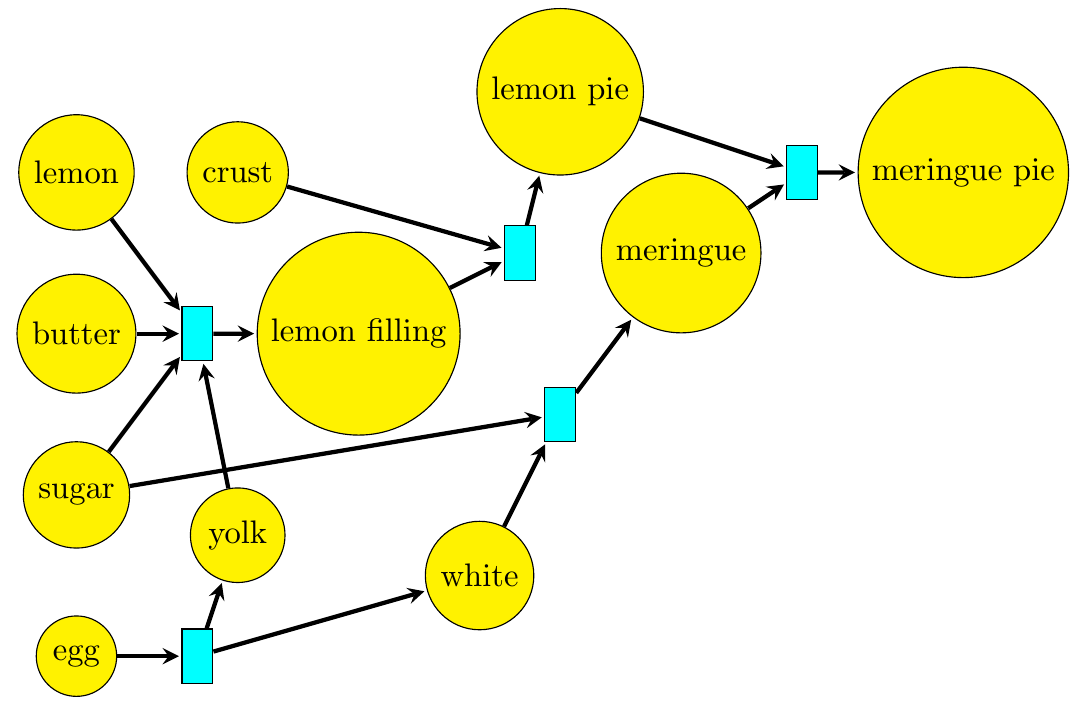While Michael was drawing his picture, I've been drawing one of my own...

I edited my lecture to point out that _reaction networks_ are just another way to provide the same information as given by _[Petri nets](https://forum.azimuthproject.org/discussion/2079/lecture-19-chapter-2-chemistry-and-scheduling/p1)!_ The reaction network I gave:

$[\textrm{egg}] \to [\textrm{yolk}] + [\textrm{white}]$
$[\textrm{lemon}] + [\text{butter}] + [\text{sugar}] + [\text{yolk}] \to [\textrm{filling}]$
$[\textrm{crust}] + [\textrm{filling}] \to [\textrm{lemon pie}]$
$[\textrm{white}] + [\textrm{sugar}] \to [\textrm{meringue}]$
$[\textrm{lemon pie}] + [\textrm{meringue}] \to [\textrm{meringue pie}]$

does the same job as this Petri net:It's just a matter of taste which one we want to use... though there are some theorems that are nice to state in terms of Petri nets and some in terms of reaction networks. You can read a lot more about both in my book:

* John Baez and Jacob Biamonte, _[Quantum Techniques for Stochastic Mechanics](https://arxiv.org/abs/1209.3632)_, Section 25.1: The Reachability Problem, World Scientific Press, 2018.